Next: Methods Up: Optimization Previous: Optimization   Contents

Definition of a minimum and a maximum

As interval coefficients may appear in the function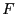we have to define what will be called a minimum or a maximum of. First we assume that there is no interval coefficients inand denote by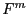the minimal or maximal value ofover a set of ranges defined for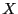and an accuracy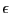with which we want to determine the extremum. The algorithm will return an interval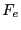as an approximation ofsuch that for a minimization problem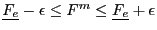and for a maximization problem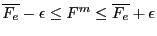. The algorithm will also return a value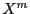forwhere the extremum occurs. If we deal with a constrained optimization problem we will have:

•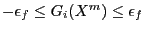•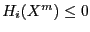or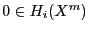and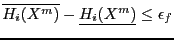•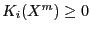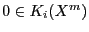and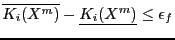where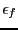has a pre-defined value. Note that if we have constraint equation of type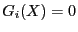the result of the optimization problem may be no more guaranteed as the constraint itself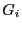may not be verified.

If there are interval coefficients in the optimum function there is not a uniquebut according to the value of the coefficient a minimal extremum value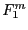and a maximal extremum value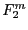. The algorithm will return in the lower bound ofan approximation ofand in the upper bound ofan approximation ofwhich verify for a minimization problem:

••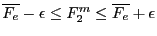Note that the width ofmay now be greater than.The algorithm will return also two solutions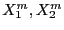forcorresponding respectively to the values of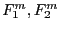. If we are dealing with a constrained optimization problem the solutions will verify the above constraint equations.

If the optimum function has no interval coefficients the algorithm may return no solution if the interval evaluation of the optimum function has a width larger than. Evidently the algorithm will also return no solution if there is no solution that satisfy all the constraints.Next: Methods Up: Optimization Previous: Optimization   Contents
Jean-Pierre Merlet 2012-12-20